# Excel IFS and SWITCH FormulaIn this tutorial, we will learn about Excel’s New Formula IFS and SWITCH.

1. IFS Formula

Here I have a table that consists of two columns score and status of a particular exam.

 <40 Fail 41 – 79 Pass >=100 Scholarship

1. If the student has scored less than 40 marks in an exam, then it will be considered as Fail

2. If the student has scored less than 79 marks in an exam, then it will be considered as Pass

3. If the student has scored more than equal to 100 marks in an exam, then it will be considered as Scholarship

So in order to calculate the score in an exam we will be applying IFS Formula:
1. Write IFS and press the Tab Key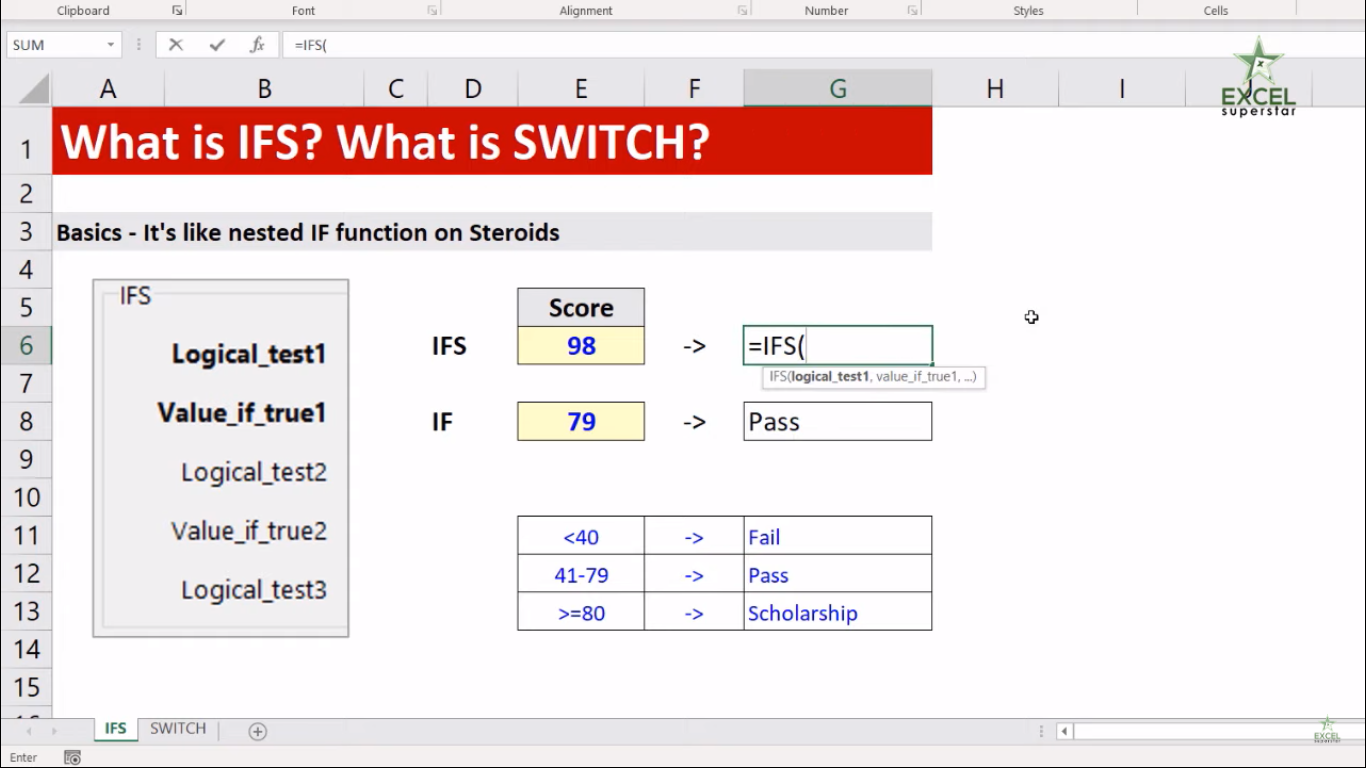Now here the formula will ask two questions

Logical_test1 – First logical test. This is a required argument and is the condition that is used by Excel to evaluate whether it is TRUE or FALSE.

Value1 – Result when logical_test1 is true

Now the first logical question will be, is the student score less than 40? If yes then

2. Click on the student score and write <40 (E6>40)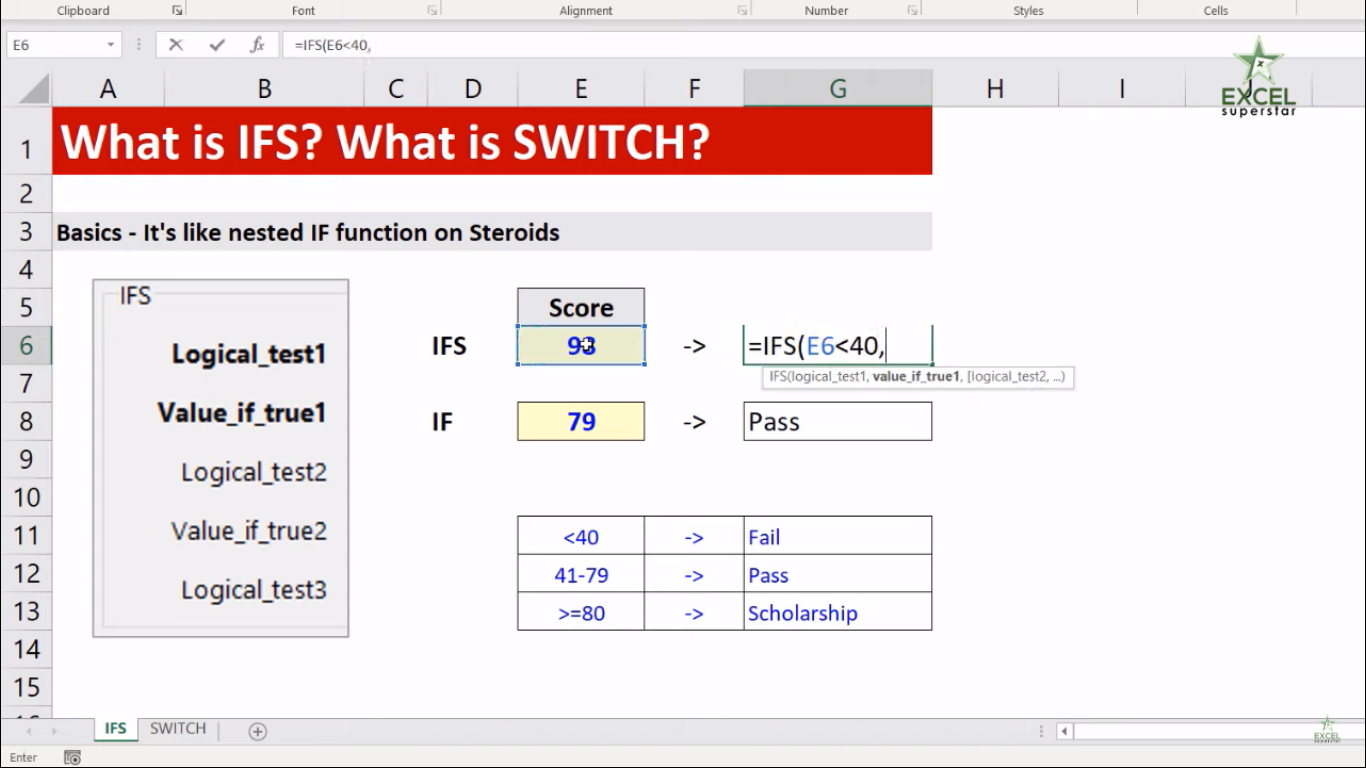3. If the score is less than 40 then write “Fail” in the message (E6>40, “Fail”)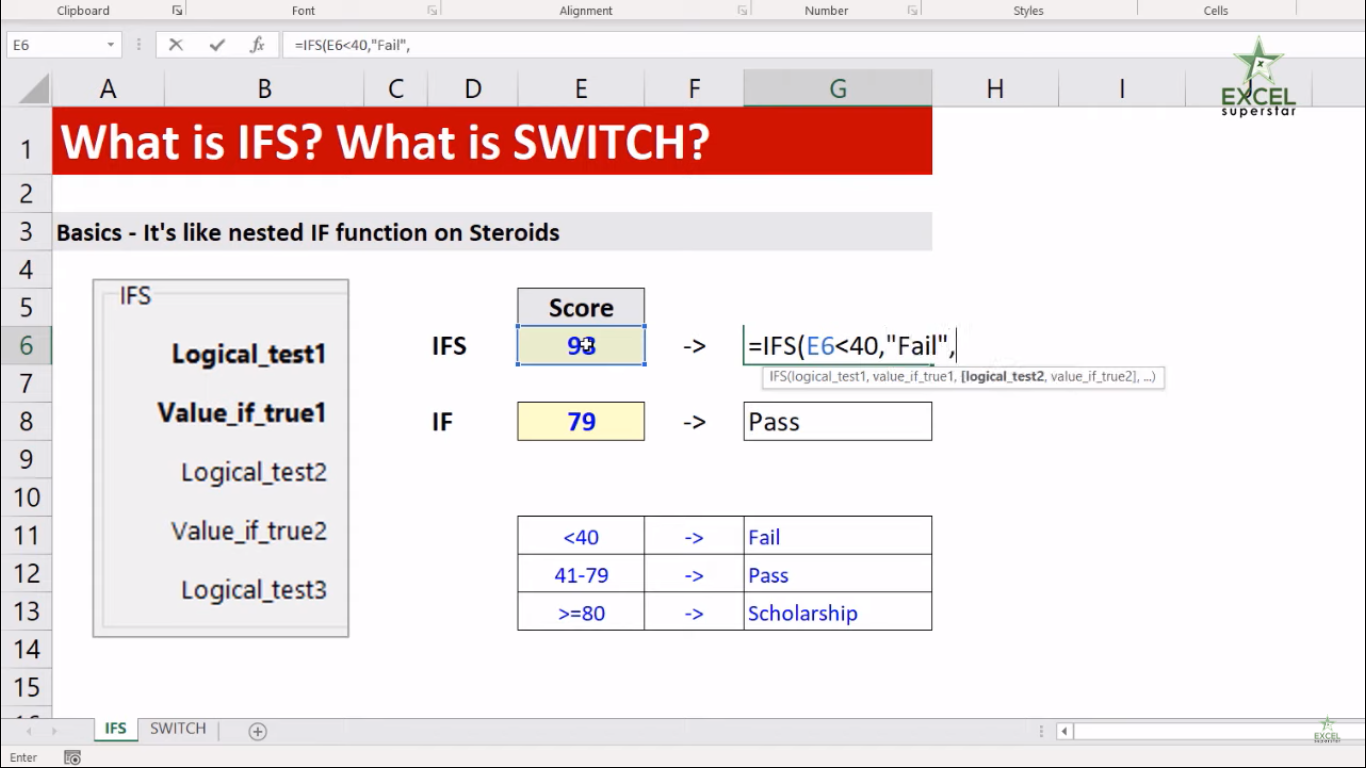The second logical question will be, is the student score less than 80? If yes then

4. Click on the student score and write <80 (E6>80)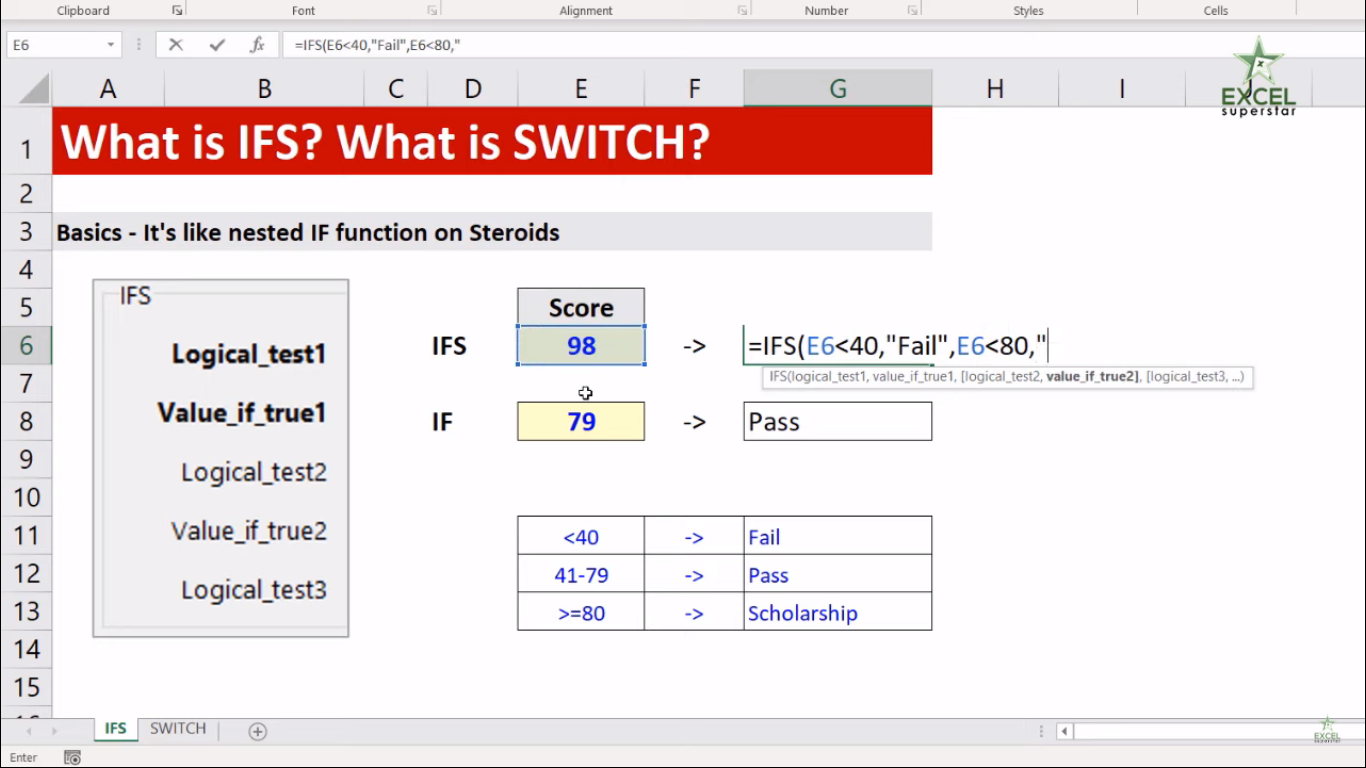5. If the score is less than 80 then write “Pass” in the message (E6>80, “Pass”)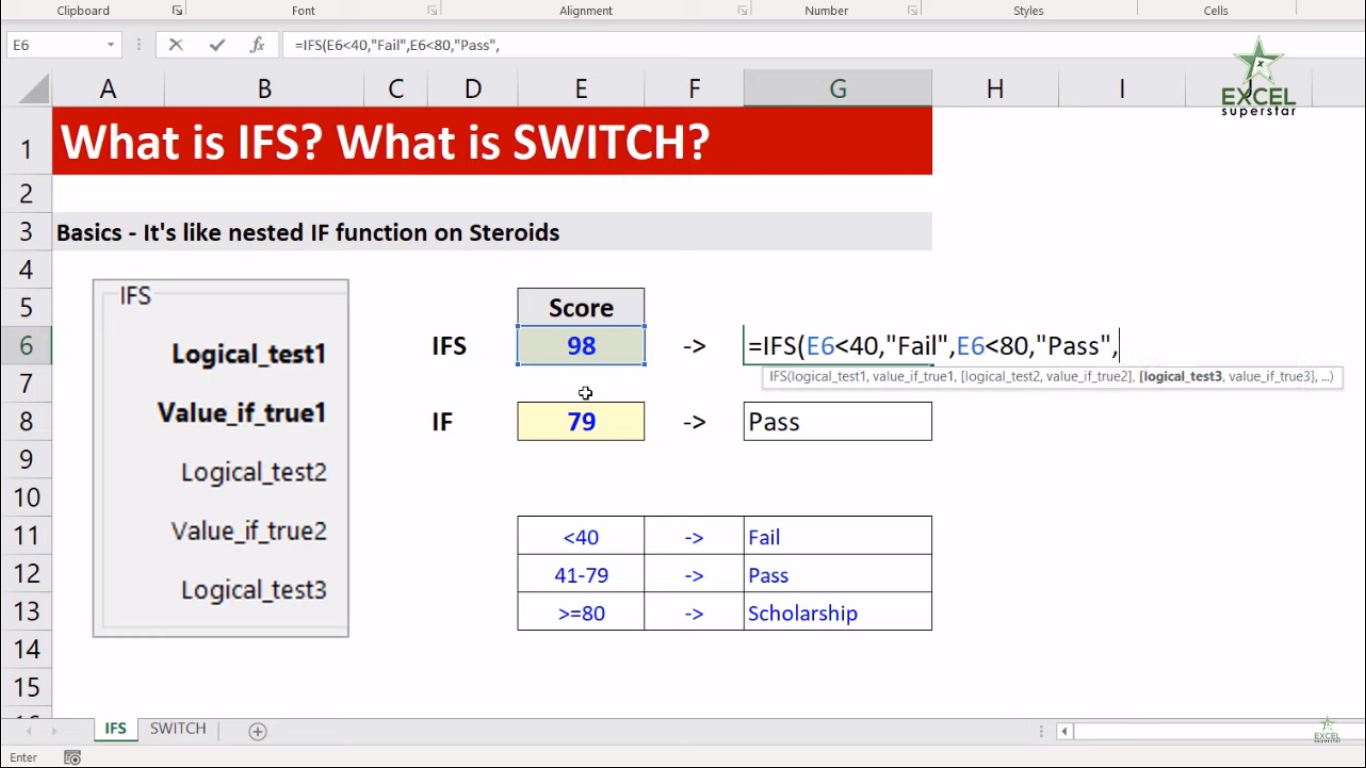The third logical question will be, is the student score less than equal to 100? If yes then

6. Click on the student score and write <=100 (E6>=100)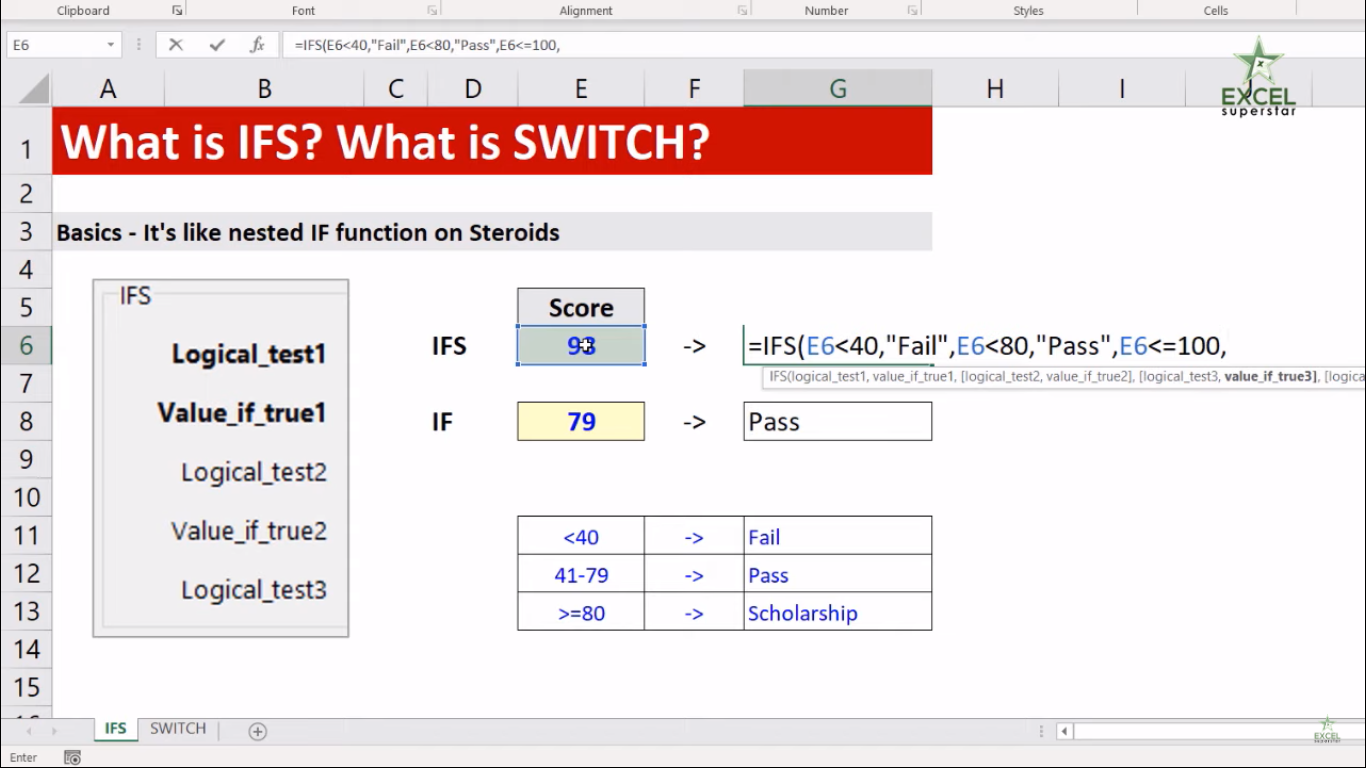7. If the score is less than equal to 100 then write “Scholarship” in the message (E6>=100, “Scholarship”)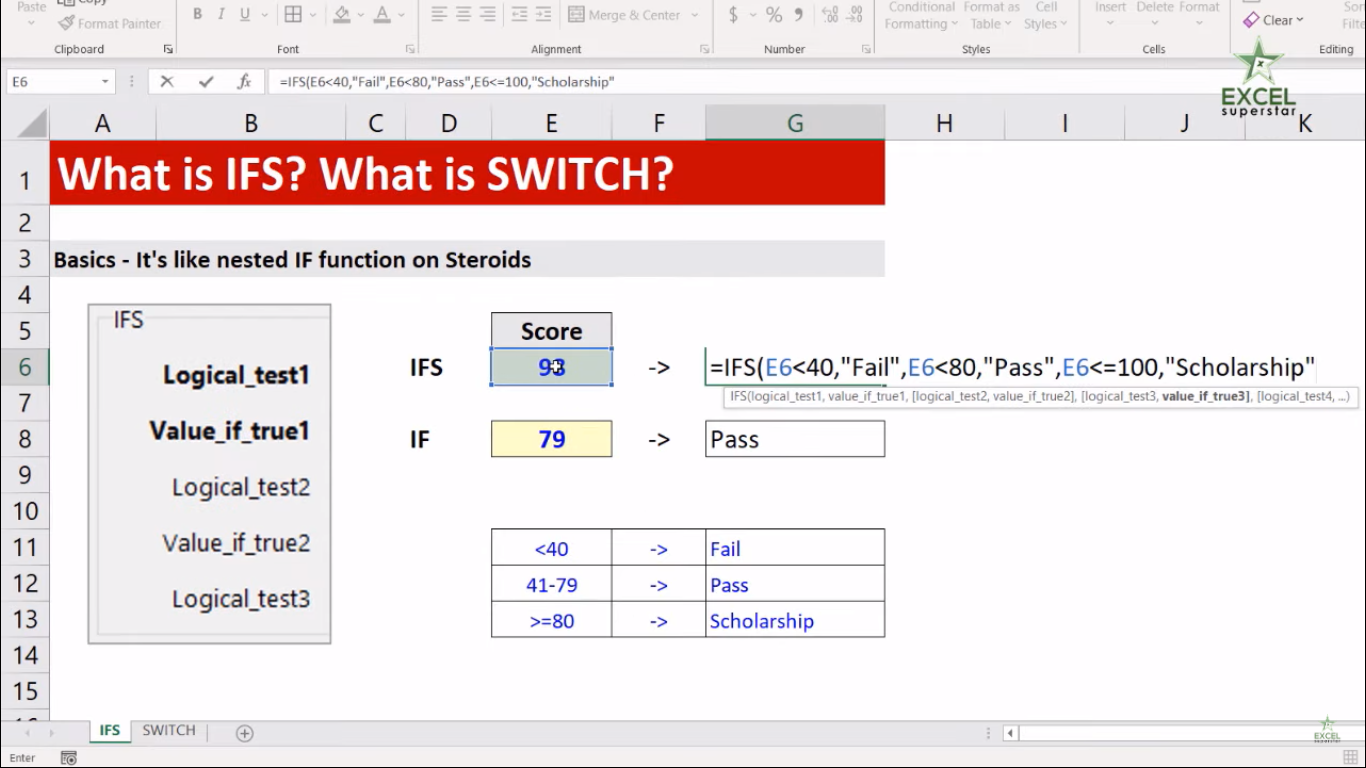8. Close the bracket and press Enter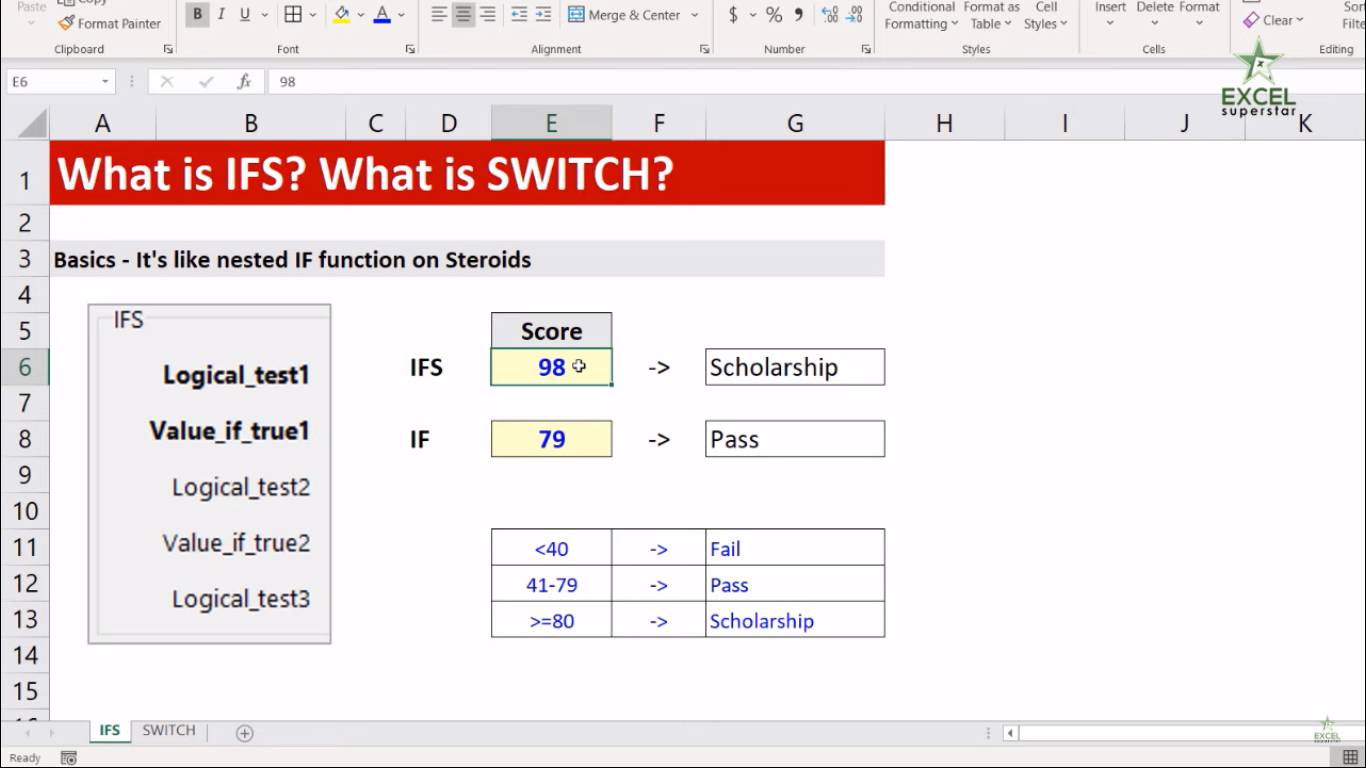9. Lastly, you will get to know the status of a score given in a cell

2. SWITCH Formula

QuestionI will write M and F in a cell. If I got M, then it will be considered as Male and, if I got F, then it will be considered as Female.

So in order to get the answer, we will be applying the SWITCH Formula:

1. Write SWITCH and press the Tab Key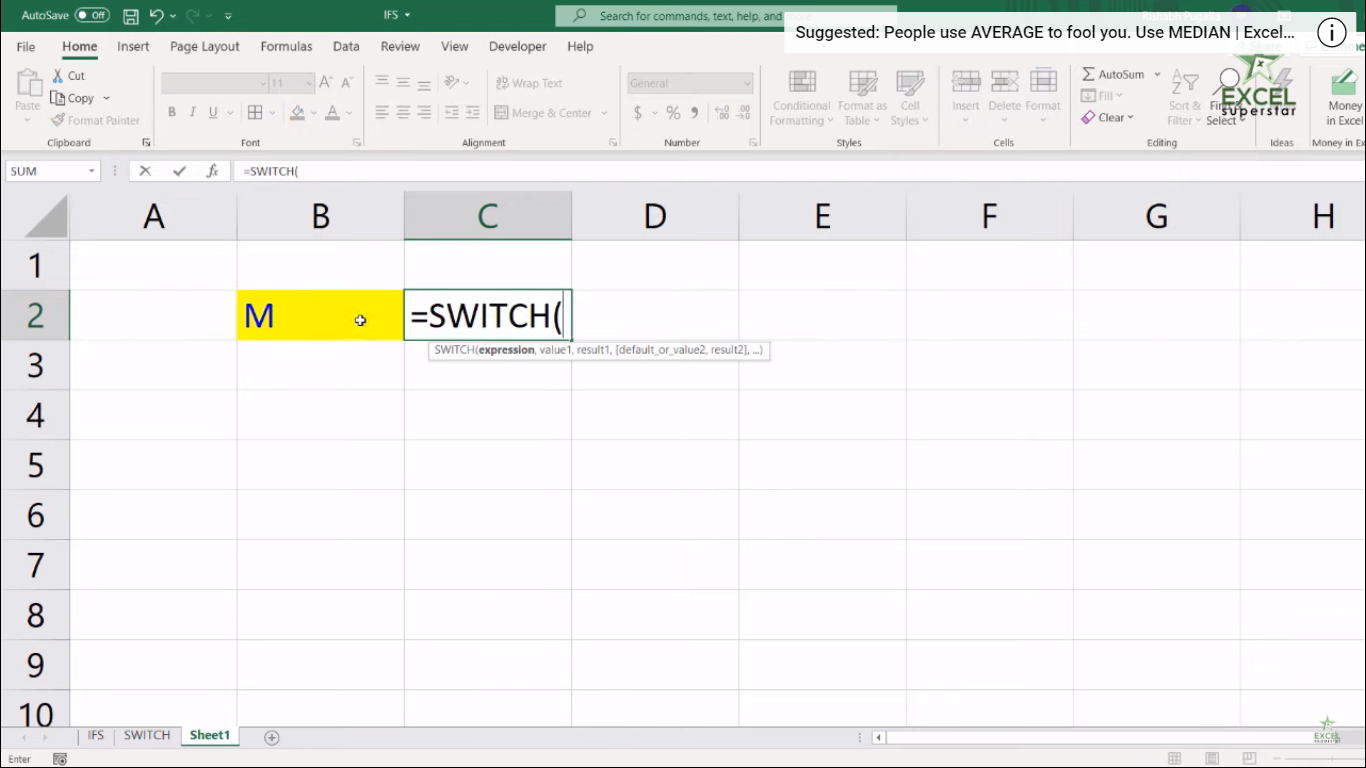Now here there are 2 parameters

1. Expression – The value or expression to match against.
2. Val1/result1 – The first value and result pair.

2. Click on the M cell to evaluate it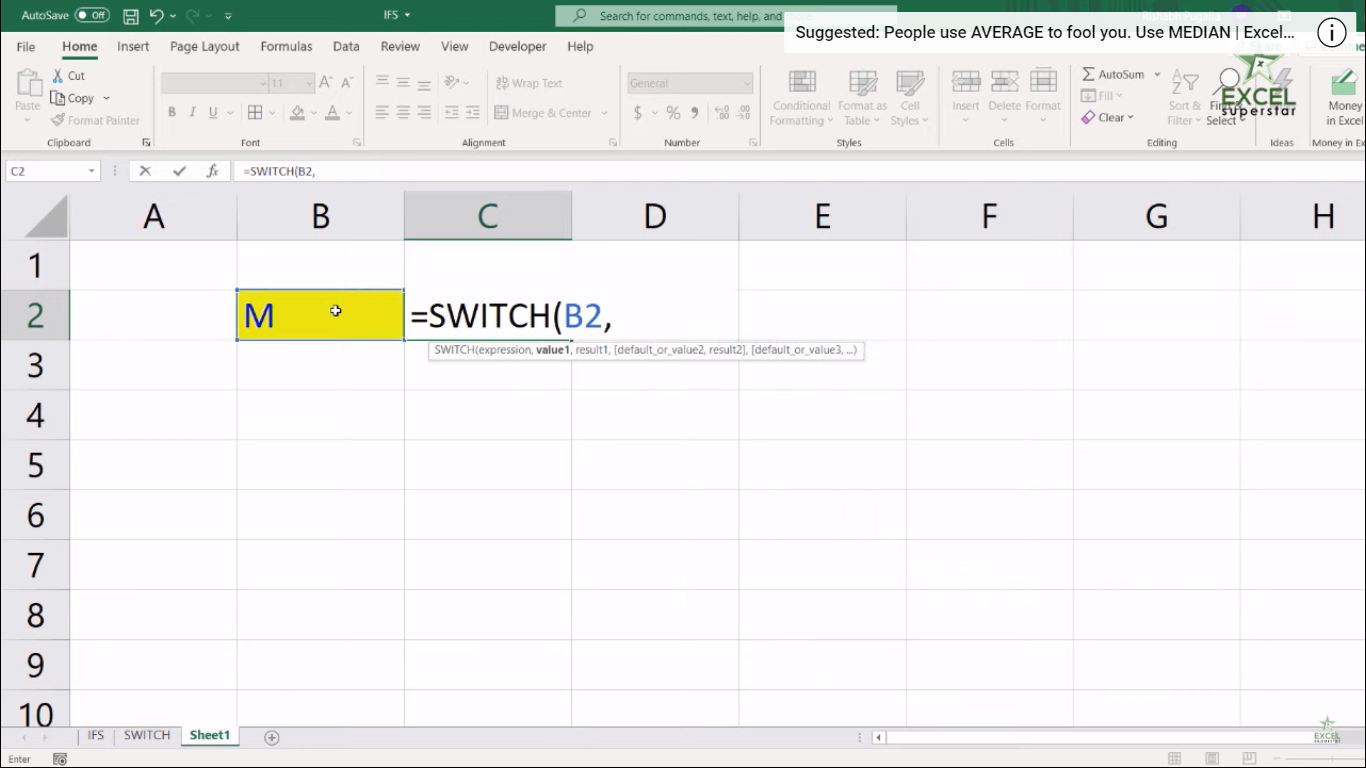3. Write “M” as value_1 and again write “Male” as a result pair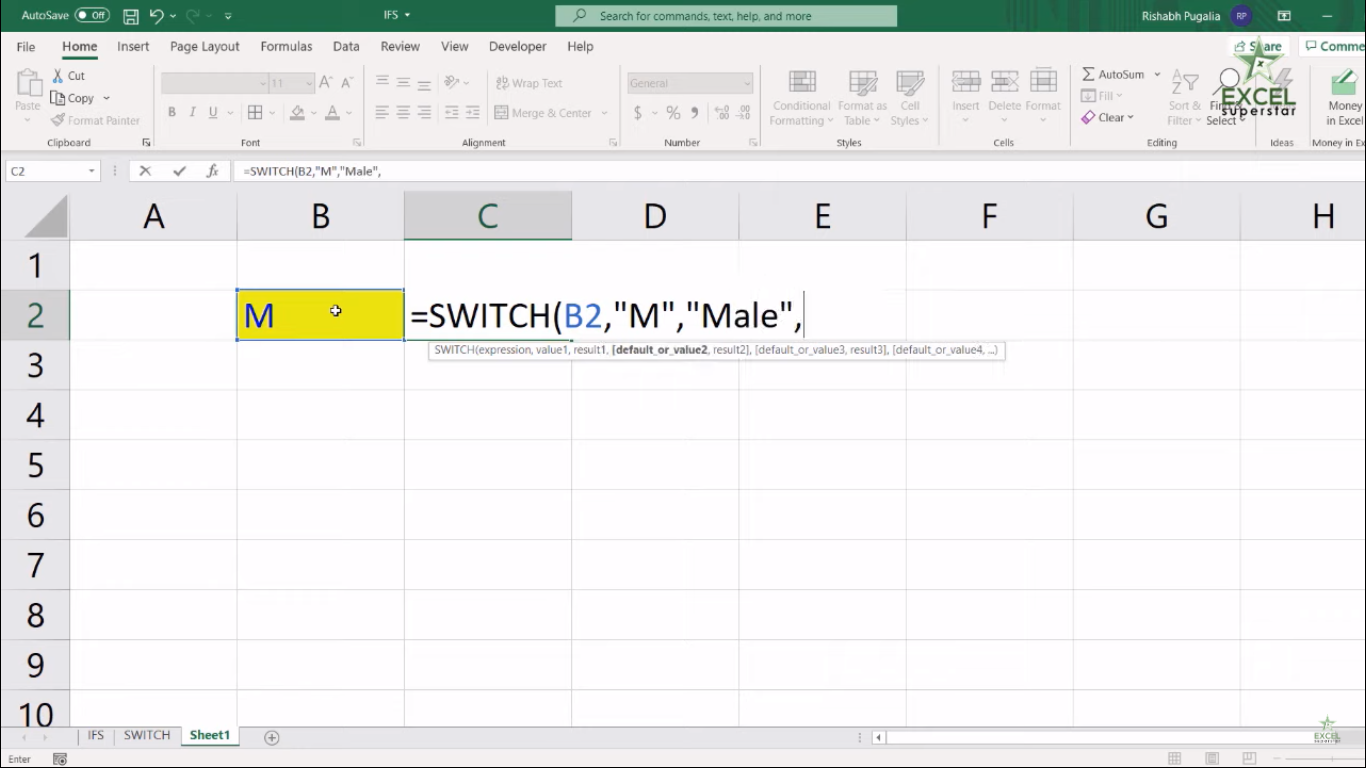4. Write “F” as value_2 and again write “Female” as a result pair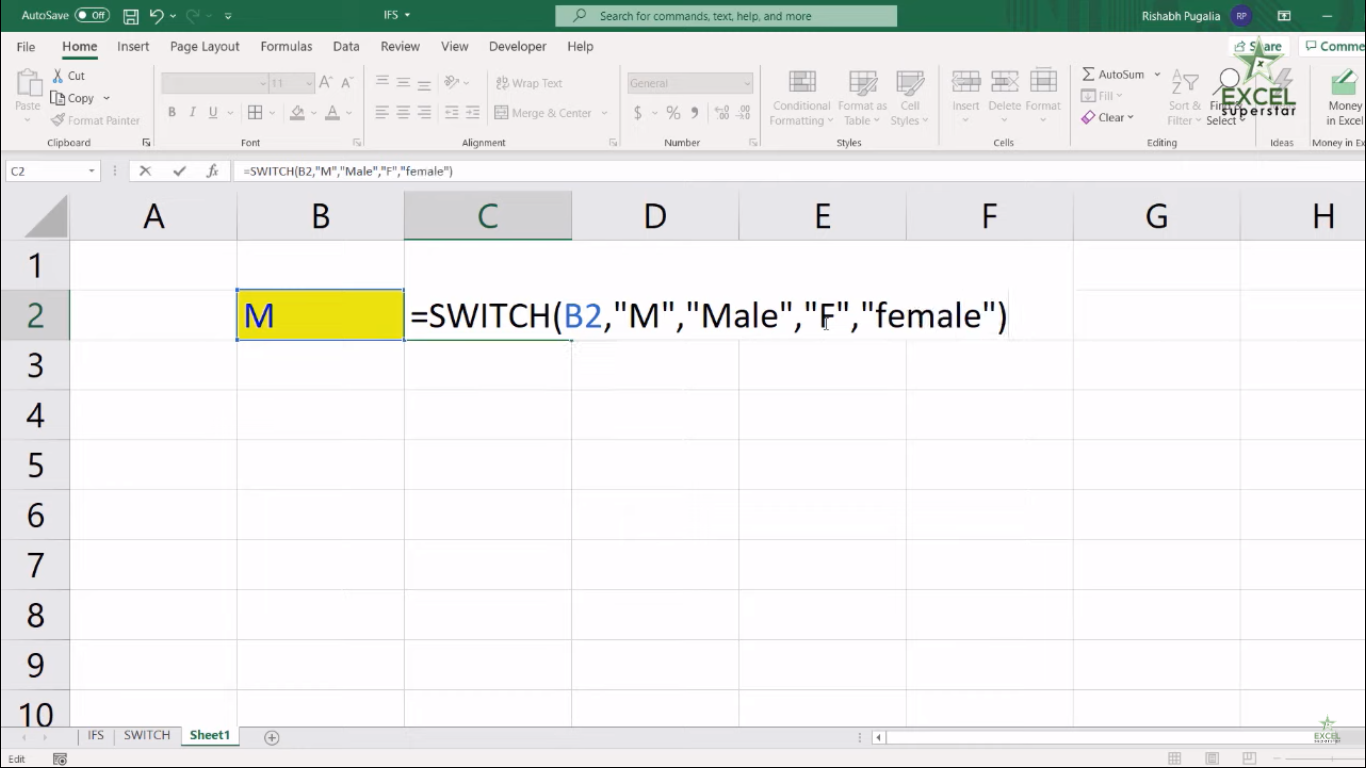5. Close the bracket and press Enter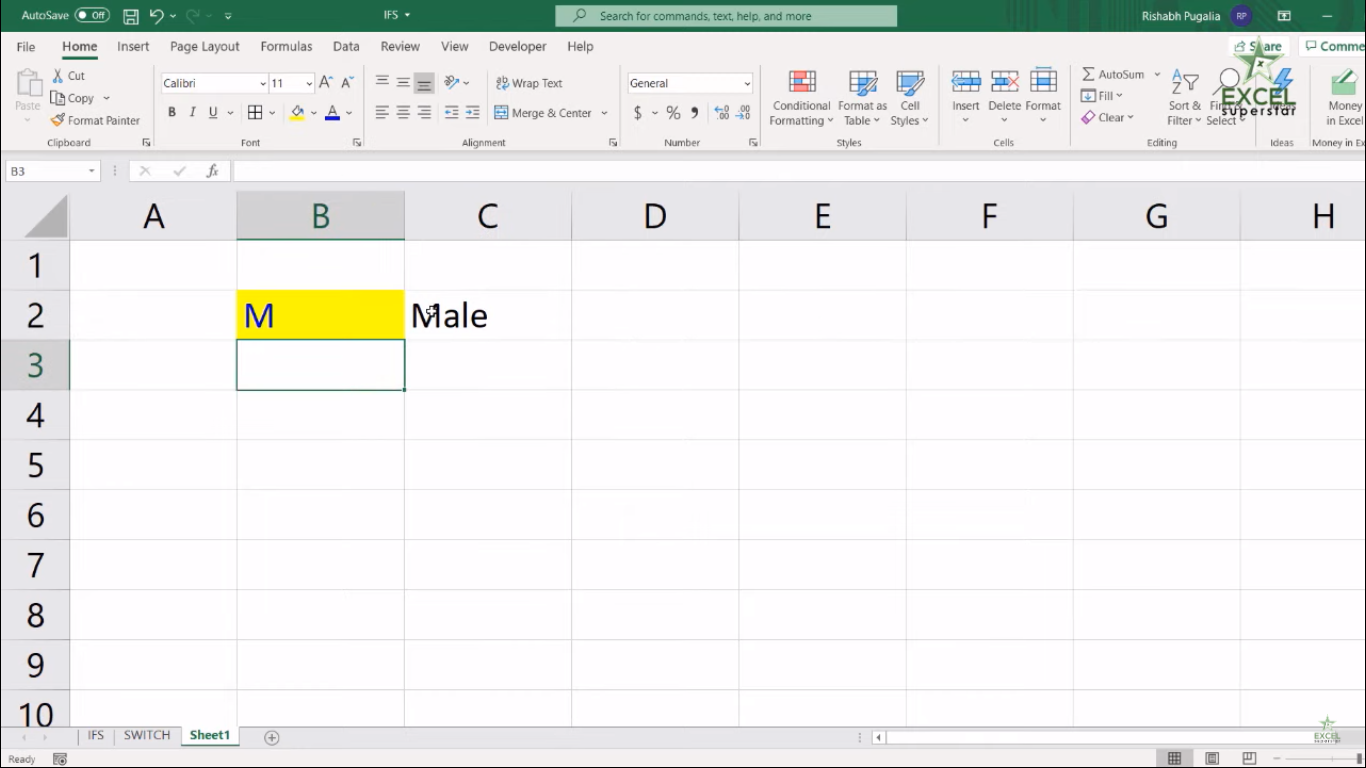6. You will get the answer M as Male also if you write F then the answer will be Female